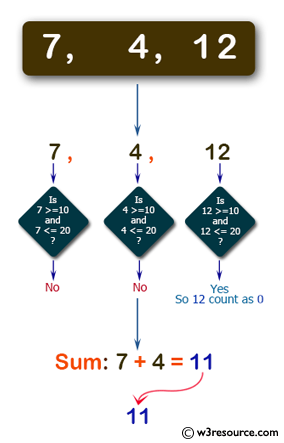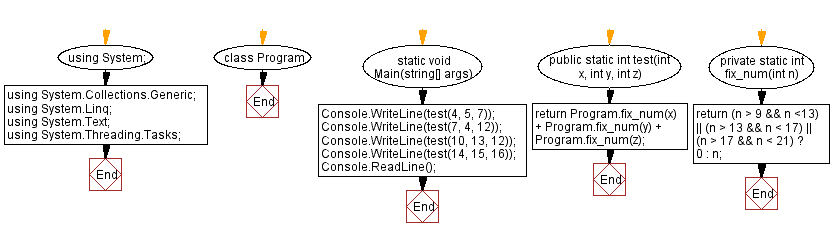﻿ C# - Compute the sum of the three given integers# C# Sharp Basic Algorithm Exercises: Compute the sum of the three given integers

## C# Sharp Basic Algorithm: Exercise-57 with Solution

Write a C# Sharp program to compute the sum of the three given integers. However, if any of the values is in the range 10..20 inclusive then that value counts as 0, except 13 and 17.

Pictorial Presentation:Sample Solution:-

C# Sharp Code:

``````using System;
using System.Collections.Generic;
using System.Linq;
using System.Text;
namespace exercises
{
class Program
{
static void Main(string[] args)
{
Console.WriteLine(test(4, 5, 7));
Console.WriteLine(test(7, 4, 12));
Console.WriteLine(test(10, 13, 12));
Console.WriteLine(test(17, 12, 18));
}
public static int test(int x, int y, int z)
{
return Program.fix_num(x) + Program.fix_num(y) + Program.fix_num(z);
}
private static int fix_num(int n)
{
return (n < 13 && n > 9) || (n > 17 && n < 21) ? 0 : n;
}
}
}
```
```

Sample Output:

```16
11
13
17```

Flowchart:C# Sharp Code Editor:

Improve this sample solution and post your code through Disqus

What is the difficulty level of this exercise?

Test your Programming skills with w3resource's quiz.

﻿